Next: Implementation Up: Solving systems of linear Previous: Solving systems of linear   Contents

Mathematical background

Let us assume that we have an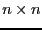interval linear system: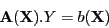(7.1)

where the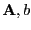elements are functions of the unknowns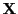. The above equation describes a family of linear system and the enclosure of this interval linear system is a box that enclose the solutions for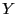of each member of the family of linear systems.

A classical scheme for finding the enclosure is to use an interval variant of the Gauss elimination scheme .

When the unknowns lie in given ranges we may compute an interval evaluation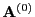of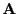and an interval evaluation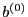of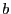. The Gauss elimination scheme may be written as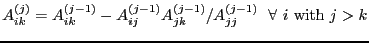(7.2)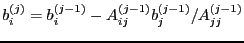(7.3)

The enclosure of the variable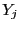can then be obtained from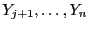by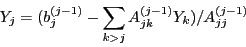(7.4)

Note that the elements at iteration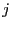can be computed only if the interval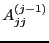does not include 0.

A drawback of this scheme is that the family of linear systems that will be obtained for all instances ofis usually a subset of the family of linear systems defined by,, as we do not take into account the dependency of the elements of. Furthermore the calculation in the scheme involves products, sums and ratio of elements ofand their direct interval evaluation again do not take into account the dependency between the elements. Hence a direct application of the Gauss scheme will usually lead to an overestimation of the enclosure.

A possible method to reduce this overestimation is to consider the system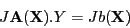where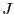is an arbitrarymatrix. The above system has the same solutions than the system (7.1) but for some matrixthe enclosure of the above interval system may be included in the enclosure obtained by the Gauss elimination scheme on the initial system: this is called a pre-conditioning of the initial system. It is usually considered that a good candidate foris the matrix whose elements are the mid-point of the ranges for the elements of.

But even with a possible goodthe dependency between the elements ofare not taken into account and hence the overestimation of the enclosure may be large.

A first possible way to reduce this overestimation is to improve the interval evaluation of,by using the derivatives of their elements with respect toand the procedure described in section 2.4.2.3. Note that the procedures necessary to compute the elements of,and their derivatives may be obtained by using the MakeF, MakeJ procedures of ALIAS-maple.

But to improve the efficiency of the procedure it must be noticed that at iterationan interval evaluation of the derivatives of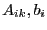with respect tomay be deduced for the derivatives of the elements computed at iteration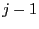. As we have the derivatives of the elements at iteration 0 we may then deduce the derivatives of the elements at any iteration and use these derivatives to improve the interval evaluation of these elements (see sections 2.4.1.1 and 2.4.2.3).Next: Implementation Up: Solving systems of linear Previous: Solving systems of linear   Contents
Jean-Pierre Merlet 2012-12-20